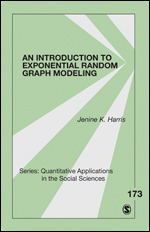Little Green Books

# An Introduction to Exponential Random Graph Modeling

This volume introduces the basic concepts of Exponential Random Graph Modeling (ERGM), gives examples of why it is used, and shows the reader how to conduct basic ERGM analyses in their own research. ERGM is a statistical approach to modeling social network structure that goes beyond the descriptive methods conventionally used in social network analysis. Although it was developed to handle the inherent non-independence of network data, the results of ERGM are interpreted in similar ways to logistic regression, making this a very useful method for examining social systems. Recent advances in statistical software have helped make ERGM accessible to social scientists, but a concise guide to using ERGM has been lacking. This book fills that gap, by using examples from public health, and walking the reader through the process of ERGM model-building using R statistical software and the statnet package.

An Introduction to Exponential Random Graph Modeling is a part of SAGE's Quantitative Applications in the Social Sciences (QASS) series, which has helped countless students, instructors, and researchers learn cutting-edge quantitative techniques. Learn more about the QASS series at http://sagepub.com/qass.

•••